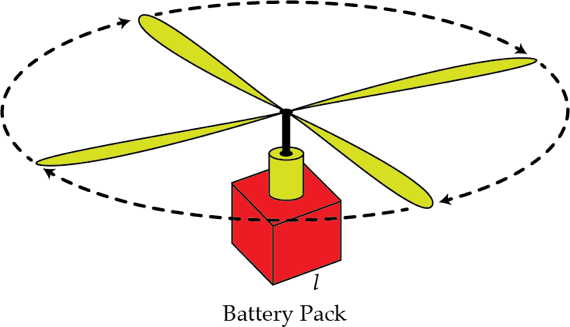# Long lasting kid fun

Toy helicopters with rechargeable batteries fly for a few minutes on a single charge. Manufacturers want to choose the right size of battery to achieve the longest flight time between charges. A larger battery stores more energy, but also increases the mass of the helicopter so it takes more energy to keep it in the air. Our question is, for a helicopter and battery type that behave as specified in the assumptions below, what is the linear size $l$ of the battery in mm that will maximize the time the helicopter can hover in place?Details and assumptions

• The acceleration of gravity is $-9.8~\mbox{m/s}^2$.
• The base mass $m_0$ of the helicopter without the battery is $50~\mbox{g}$.
• The battery is a uniform cube with side length $l$ and density $\rho= 4000 ~\mbox{kg/m}^3$.
• The total energy contained in the battery does not quite scale as the volume does, but instead scales as $l^{2.7}$.
• The emitted voltage from the battery is constant and does not change with $l$. All that changes is how long the battery lasts. Therefore the rotors spin and push air in the same way no matter what the battery size is.
• Assume the mass of the air pushed by the rotors is a constant value, independent of rotor speed. In an actual helicopter the mass increases with speed, effectively from Bernoulli's equation, but that's more complication than we want to go into.
×

Problem Loading...

Note Loading...

Set Loading...